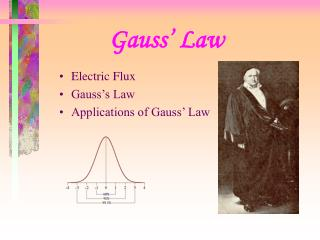DownloadDownload PresentationGauss’ Law

# Gauss’ Law

Download Presentation## Gauss’ Law

- - - - - - - - - - - - - - - - - - - - - - - - - - - E N D - - - - - - - - - - - - - - - - - - - - - - - - - - -
##### Presentation Transcript

1. Gauss’ Law • Electric Flux • Gauss’s Law • Applications of Gauss’ Law

2. Gauss’ Law • Here are a number of charge arrangements. really 3-dimensional • Is there any connection between the number of field lines through a surface and the charge enclosed.

3. Gauss’ Law electric field • Electrical Flux • vector area • Magnitude is common old area • Direction is normal to area

4. f = 0 f = -f0 f = f0 f = 0 E Gauss’s Law • What about the flux through a closed surface? f = 0 • What about this surface? f = 0 ???? yes

5. Gauss’ Law • Gauss’s law In general this is a hard integral to do. There are some simple cases where symmetry helps us perform the integral. Let’s look at the point charge!

6. Gauss’ Law • Gauss’ law for a point charge What can we say about E and dA? What can you say about the magnitude of E on the surface? They are in the same direction so cos q = cos 0 = 1 E depends only on r so constant

7. Gauss’ Law What is ?

8. Gauss’ Law • Electric Flux

9. |E| r a Gauss’s Law Q • What is the electric field for a charged shell What is charge inside Gaussian surface 1? What is flux through the surface? What is the electric field on the surface? What is electric field inside surface? Gaussian surfaces 1 and 2 Q = 0 f = 0 from Gauss’s law E = 0 from Gauss’s law E = 0 from symmetry

10. |E| 1/r2 r a Gauss’ Law Q • What is the electric field for a charged shell What is charge inside Gaussian surface 2? What is flux through the surface? What is the electric field on the surface? What is electric field outside surface? Gaussian surfaces 1 and 2 Q f = Q/e0 from Gauss’s law E = kQ/a2 from Gauss’s law E = kQ/r2 from symmetry

11. Gauss’ Law • We can also use Gauss’s Law to find the electric field for a charge spread out inside a sphere.

12. Gauss’ Law • Gauss’s Law can be used with different surfaces like a cylinder or cap, but we do not have time to explore these.

13. Gauss’ Law • What about a conductor? The electric field is zero inside a conductor in electrostatic equilibrium. Near the surface of a conductor in electrostatic equilibrium and with a net charge the electric field is perpendicular to the surface. If a conductor in electrostatic equilibrium carries a net charge, all excess charge resides on the conductor surface.

14. Gauss’ Law • What about a conductor. The electric field is perpendicular to the surface of a conductor. Since all the charge is on the surface the electric field is zero inside a conductor.